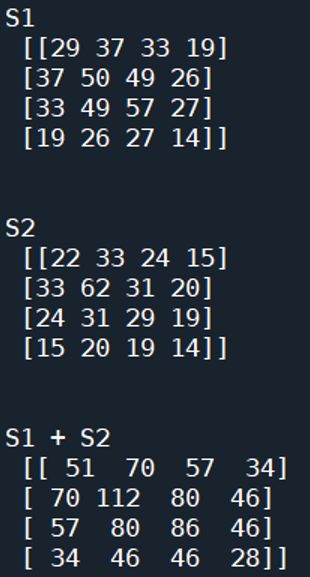# Sum of Symmetric Matrices | Linear Algebra using Python

Linear Algebra using Python | Sum of Symmetric Matrices: Here, we are going to learn about the sum of symmetric matrices and its implementation in Python.
Submitted by Anuj Singh, on June 13, 2020

Prerequisite:

Here, we will learn how to create two symmetric matrices and will add them? Adding two symmetric matrices results in a symmetric matrix.

S0 = S1 + S2

Note: The dimensions of S1 and S2 are same!

Example:## Python code to find sum of symmetric matrices

```# Linear Algebra Learning Sequence
# Addition of two symmetric Matrix

import numpy as np

M1 = np.array([[2,3,4], [3,5,4], [2,7,2], [1,3,2]])
M2 = np.array([[2,3,3], [3,2,7], [3,4,2], [3,2,1]])

S1 = np.matmul(M1,M1.T)
S2 = np.matmul(M2,M2.T)

print('\n\nS1\n', S1)
print('\n\nS2\n', S2)

print('\n\nS1 + S2\n', S1+S2)
```

Output:

```
S1
[[29 37 33 19]
[37 50 49 26]
[33 49 57 27]
[19 26 27 14]]

S2
[[22 33 24 15]
[33 62 31 20]
[24 31 29 19]
[15 20 19 14]]

S1 + S2
[[ 51  70  57  34]
[ 70 112  80  46]
[ 57  80  86  46]
[ 34  46  46  28]]
```

Preparation

What's New

Top Interview Coding Problems/Challenges!